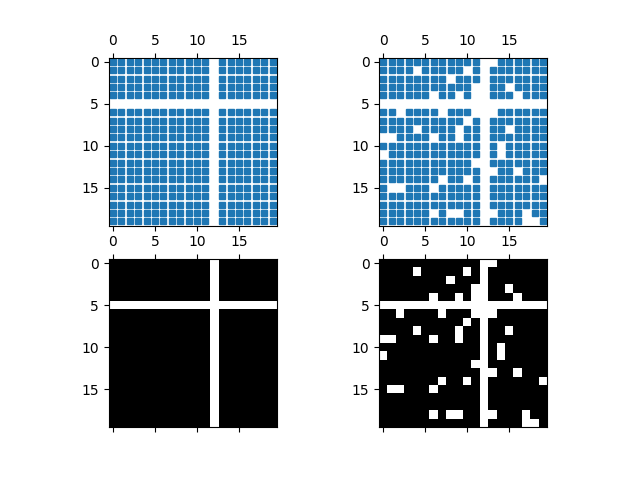# Spy Demos#

Plot the sparsity pattern of arrays.

import matplotlib.pyplot as plt
import numpy as np

# Fixing random state for reproducibility
np.random.seed(19680801)

fig, axs = plt.subplots(2, 2)
ax1 = axs[0, 0]
ax2 = axs[0, 1]
ax3 = axs[1, 0]
ax4 = axs[1, 1]

x = np.random.randn(20, 20)
x[5, :] = 0.
x[:, 12] = 0.

ax1.spy(x, markersize=5)
ax2.spy(x, precision=0.1, markersize=5)

ax3.spy(x)
ax4.spy(x, precision=0.1)

plt.show()References

The use of the following functions, methods, classes and modules is shown in this example:

Gallery generated by Sphinx-Gallery